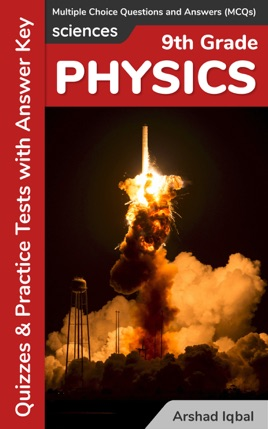• \$4.99

## Publisher Description

Grade 9 Physics Multiple Choice Questions and Answers (MCQs): Quizzes & Practice Tests with Answer Key provides mock tests for competitive exams to solve 765 MCQs. "Grade 9 Physics MCQ" PDF to download helps with theoretical, conceptual, and analytical study for self-assessment, career tests. Grade 9 Physics Quizzes, a quick study guide can help to learn and practice questions for placement test preparation.
Grade 9 Physics Multiple Choice Questions and Answers (MCQs) PDF to download is a revision guide with a collection of trivia quiz questions and answers PDF on topics: Dynamics, gravitation, kinematics, matter properties, physical quantities and measurement, thermal properties of matter, transfer of heat, turning effect of forces, work and energy to enhance teaching and learning.
Grade 9 Physics Quiz Questions and Answers PDF also covers the syllabus of many competitive papers for admission exams of different schools from physics textbooks on chapters:
Dynamics MCQs: 87 Multiple Choice Questions. Gravitation MCQs: 48 Multiple Choice Questions. Kinematics MCQs: 100 Multiple Choice Questions. Matter Properties MCQs: 96 Multiple Choice Questions. Physical Quantities and Measurement MCQs: 112 Multiple Choice Questions. Thermal Properties of Matter MCQs: 107 Multiple Choice Questions. Transfer of Heat MCQs: 52 Multiple Choice Questions. Turning Effect of Forces MCQs: 62 Multiple Choice Questions. Work and Energy MCQs: 101 Multiple Choice Questions.
"Dynamics MCQ" PDF covers quiz questions about dynamics and friction, force inertia and momentum, force, inertia and momentum, Newton's laws of motion, friction, types of friction, and uniform circular motion.
"Gravitation MCQ" PDF covers quiz questions about gravitational force, artificial satellites, g value and altitude, mass of earth, variation of g with altitude.
"Kinematics MCQ" PDF covers quiz questions about analysis of motion, equations of motion, graphical analysis of motion, motion key terms, motion of free falling bodies, motion of freely falling bodies, rest and motion, scalars and vectors, terms associated with motion, types of motion.
"Matter Properties MCQ" PDF covers quiz questions about kinetic molecular model of matter, Archimedes principle, atmospheric pressure, elasticity, Hooke’s law, kinetic molecular theory, liquids pressure, matter density, physics laws, density, pressure in liquids, principle of floatation, and what is pressure.
"Physical Quantities and Measurement MCQ" PDF covers quiz questions about physical quantities, measuring devices, measuring instruments, basic measurement devices, introduction to physics, basic physics, international system of units, least count, significant digits, prefixes, scientific notation, and significant figures.
"Thermal Properties of Matter MCQ" PDF covers quiz questions about change of thermal properties of matter, thermal expansion, thermal physics, state, equilibrium, evaporation, latent heat of fusion, latent heat of vaporization, specific heat capacity, temperature and heat, temperature conversion, and thermometer.
"Transfer of Heat MCQ" PDF covers quiz questions about heat, heat transfer and radiation, application and consequences of radiation, conduction, convection, general physics, radiations and applications, and thermal physics.
"Turning Effect of Forces MCQ" PDF covers quiz questions about torque or moment of force, addition of forces, like and unlike parallel forces, angular momentum, center of gravity, center of mass, couple, equilibrium, general physics, principle of moments, resolution of forces, resolution of vectors, torque, and moment of force.
"Work and Energy MCQ" PDF covers quiz questions about work and energy, efficiency, forms of energy, inter-conversion of energy, kinetic energy, sources of energy, potential energy, power, major sources of energy, and efficiency.